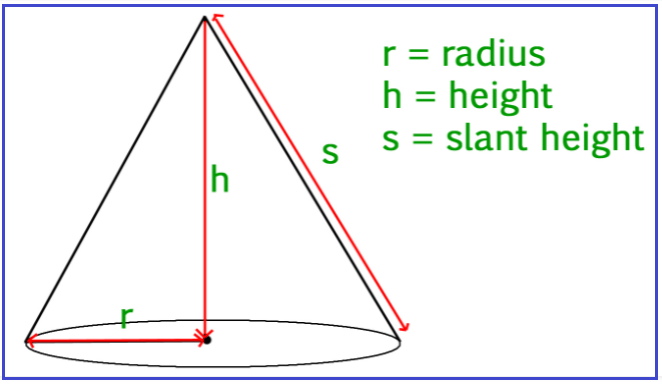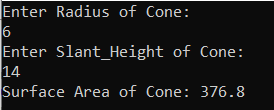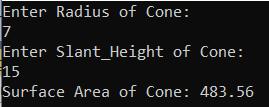# Surface Area of Cone in C#

## Surface Area of Cone in C# with Examples

In this article, I am going to discuss how to calculate the Surface Area of Cone in C# with Examples. Please read our previous article, where we discussed How to Calculate the Volume of Cylinder in C#. Here, in this article, first, we will discuss what is Cone, then we will discuss how to calculate the Surface Area of Cone and finally, we will see how to implement this in C#.

##### What is a Cone?

According to Wikipedia, A cone is a three-dimensional geometric shape that tapers smoothly from a flat base to a point called the apex or vertex. A cone is formed by a set of line segments, half-lines, or lines connecting a common point, the apex, to all of the points on a base that is in a plane that does not contain the apex.##### Surface Area of Cone:

A cone is a three-dimensional geometric figure that has a flat surface and a surface pointed towards the top. The pointed end of the cone is called the apex, whereas the flat surface is called the base. The total surface area will be equal to the sum of its curved surface area and circular base area.

Total Surface Area =πr2+πrs
=πr(r+s)
Where r is the base radius of the cone and s is the slant height of a cone

###### Example:

total surface area =πr2+πrs
=πr(r+s)
=3.14*5(5+13)
=282.6

##### Logic to Calculate the Surface Area of Cone in C#

To find the Surface Area of Cone, we need to follow the below steps

1. We will define Radius, Slant_Height, and SurfaceArea variables.
2. Then we will assign the value of Radius and Slant_Height.
3. Then we will calculate the surface area of the cone by using this formula πr2+πrs, where, π =3.14
4. And the resulting output will store in the SurfaceArea variable.
##### Example: Surface Area of Cone Program in C#.

The following C# Program will calculate the Surface Area of Cone.

```using System;
public class SurfaceAreaOfCone
{
public static void Main()
{
float Slant_Height = 13;
double PI = 3.14;

Console.WriteLine("Surface Area of Cone: " + SurfaceArea);
}
}
```

Output: Surface Area of Cone: 282.6

##### Example: Taking input from the user

In the following C# program, we will take the cone radius and slant height from the user and then calculate the Surface Area and print it on the console.

```using System;
public class SurfaceAreaOfCone
{
public static void Main()
{
Console.WriteLine("Enter Slant_Height of Cone:");

double PI = 3.14;

Console.WriteLine("Surface Area of Cone: " + SurfaceArea);
}
}
```
###### Output:##### C# Code (Using function)

In the below example, we have created one method to calculate the total surface area of the cone.

```using System;
public class SurfaceAreaOfCone
{
public static void Main()
{
Console.WriteLine("Enter Slant_Height of Cone:");

double PI = 3.14;
double SurfaceArea = CalculateSurfaceArea(Radius, Slant_Height, PI);
Console.WriteLine("Surface Area of Cone: " + SurfaceArea);